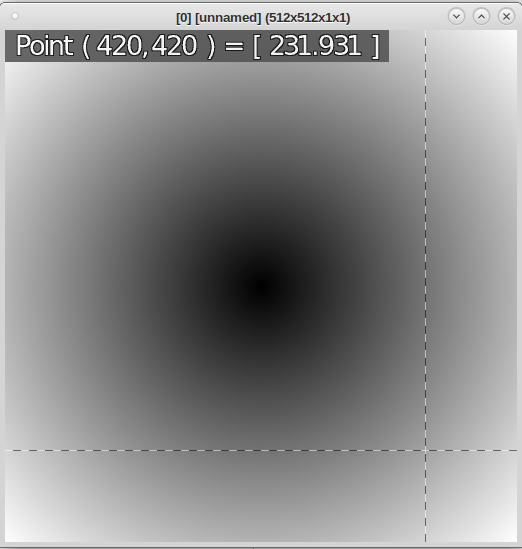# How to create a halo?

Hallo,

I want to create a halo using the cli.

I have a picture which consists of a single matrix (i.e. the spectrum c is 1).
The values of the matrix either 0 or 1.

I want to compute a new matrix as follows:
The value at some entry [i,j] of the new matrix is the (euclidian)
distance between i,j and the closest 0-entry of the original matrix.

Best regards, Daniel

Try `distance`
Tutorial: -distance
Reference: -distance Reference

``````gmic 512,512,1,1 set 1,50%,50% -distance. 1
````````````In : np.sqrt(2*(420 - 256)**2)
Out: 231.93102422918759``````

@grosgood Thank you very much, I will within the next days.

DanielDD